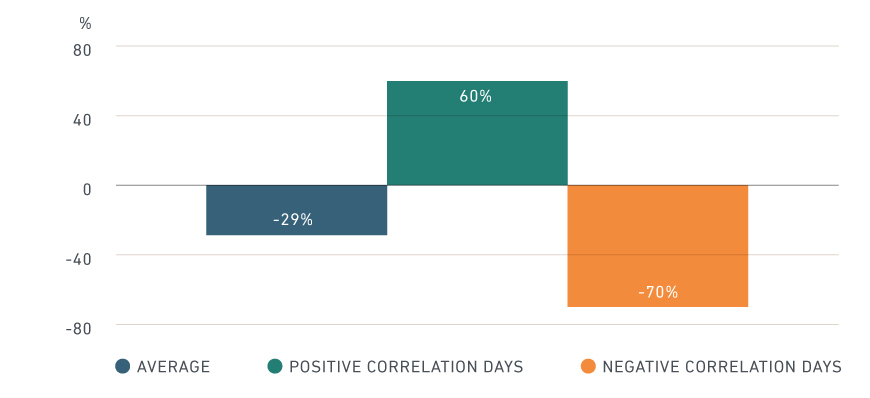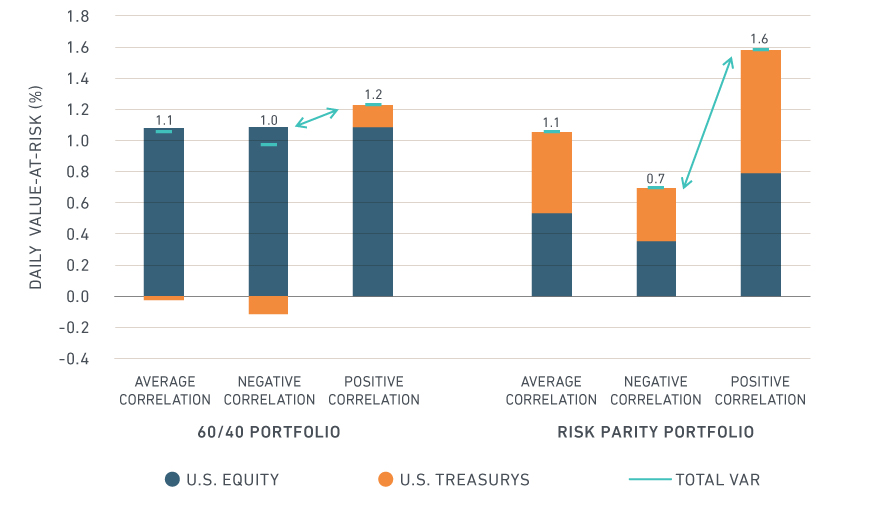## Author DetailsThomas Verbraken
Executive Director, MSCI Research

# The risk in risk-parity strategiesThe relationship between bonds and equities – one of the most-closely-followed correlation measures in financial markets – may be especially important to investors who employ a risk-parity approach. In our analysis, as the bond-equity correlation turned strongly positive, the effect on risk-parity portfolios (allocated based on equal contribution of risk) was much greater than that on traditional 60/40 equity/bond portfolios (allocated based on asset type).

##### Measuring the effects of correlation shifts

To assess the impact of the bond-equity correlation on portfolio risk, we estimate the bond-equity correlation based on the days when bonds moved opposite to equities and when they moved together between January 2013 and January 2019. This allows us to test extreme assumptions for the bond-equity correlation, effectively changing it from nearly -70% to +60%. The full-period average correlation estimate was roughly -30%.

###### Bond-equity correlations estimatesAverage bond-equity correlation over the entire period (Jan. 1, 2013 to Jan. 1, 2019) as well as the correlation estimate based on days when bonds and equities moved together or opposite.

Next, we assess the risk of a traditional 60/40 and a risk-parity portfolio1 for each of the three correlation estimates in the exhibit above during the same period.2 The 60/40 portfolio’s risk was largely driven by equity risk, with bonds acting as a (small) cushion when the correlation is negative. Therefore, the difference in risk of a 60/40 portfolio between the two extreme correlation assumptions was marginal. The risk-parity portfolio, on the other hand, heavily over-weighted bonds to obtain an equal risk contribution from both asset classes. For such portfolios, the total risk more than doubled when going from a strongly negative to a large positive correlation.

###### Bond-equity correlation changes had greater effect on risk-parity portfoliosOne-day 99% value-at-risk for a 60/40 and a risk-parity portfolio based on U.S. equity and Treasury bonds for varying degrees of historical correlation and volatility.

Our analysis suggests that the extent to which a changing bond-equity correlation affected a portfolio depended to a large extent on the asset allocation approach, as risk-parity portfolios were more vulnerable than equity-heavy 60/40 portfolios.

The author thanks Monika Szikszai for her contributions to this post.

1We proxy the U.S. equity market with MSCI USA and U.S. Treasury’s with ICE BofAML US Treasury Index (G0Q0), used with permission. We construct a risk-parity portfolio, in which bonds and equities contribute equally to risk, based on the average volatility and correlation estimates. We apply leverage to ensure the risk-parity portfolio has the same level of risk as the 60/40 portfolio (under the average correlation assumption).

2We calculate one-day 99% Value-at-Risk (VaR) for the two portfolios. MSCI’s RiskManager allows decoupling volatility and correlation estimation, effectively using different historical data and weighting schemes to estimate both numbers. The risk settings used in this blog post and an implementation guide are available on the support site.